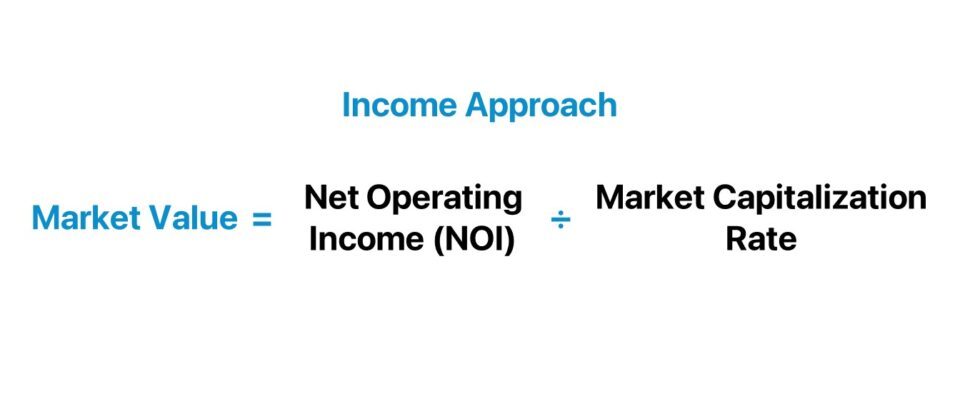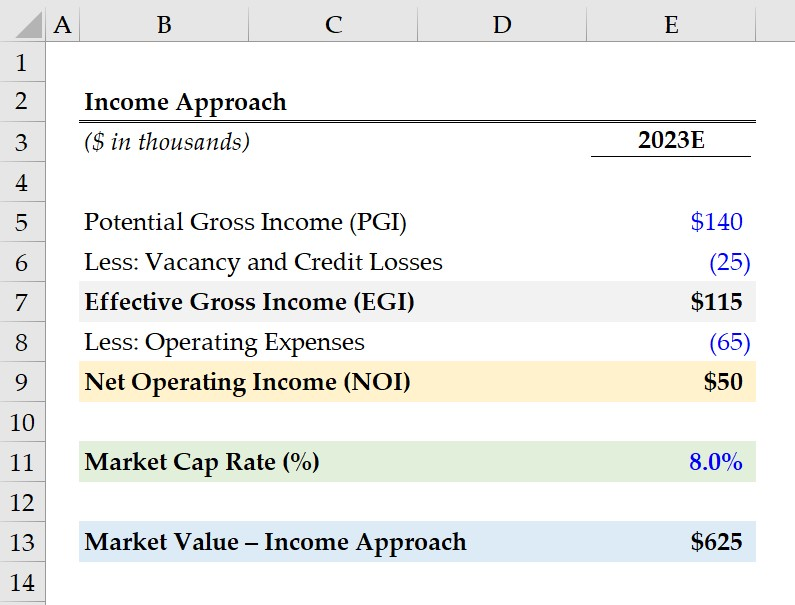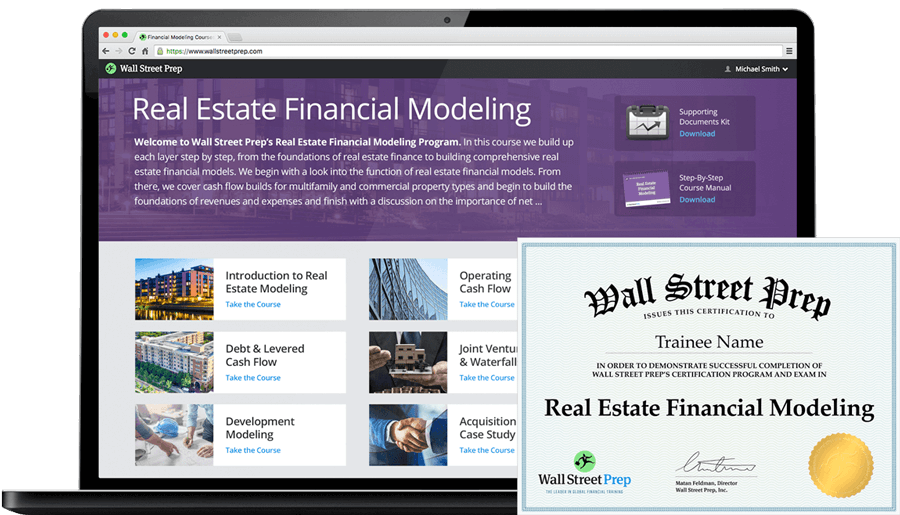Welcome to Wall Street Prep! Use code at checkout for 15% off.# Income Approach

Guide to Understanding the Income Approach to Value ("Capitalization")## How to Calculate Income Approach in Real Estate Valuation?

The income approach, under the specific context of real estate valuation, is a method used by appraisers to determine a property’s market value based on its income.

The income approach, or “capitalization approach”, states that the value of an investment property is a function of the quality and quantity of the income it is expected to generate.

The estimation of an income-producing property is performed via capitalization, where the present income of a property is adjusted by an appropriate rate of return to estimate the true value of an income stream.

Because the capitalization approach is the process of converting an anticipated stream of income into a market value indicator, the term is often used interchangeably with the income approach.

• Net Operating Income (NOI) → The net operating income (NOI) is a real estate metric representing a property’s operating income, minus the operating expenses incurred in a given period. The NOI is most frequently calculated on an annual basis, and thus reflects the future revenue (and profit potential) of a property. NOI is unaffected by discretionary financing decisions.
• Capitalization Rate → The capitalization rate (or “cap rate”) is a fundamental real estate metric calculated by dividing the net operating income (NOI) of a property by its asset value. The cap rate concept can be thought of as the implied yield on a property investment across one year.## How Does the Income Approach Appraisal Work?

The income approach is among one of the three primary methodologies that appraisers utilize to make an appraisal.

The two other methods, aside from the income approach, are the following:

The income approach treats the net operating income (NOI) of a property as a perpetuity, i.e. a constant stream of cash flows discounted using an appropriate required rate of return based on the market.

The aforementioned hurdle rate is formally termed the market capitalization rate, or “market cap rate”. The market cap rate is the rate of return used as of the present date to capitalize future income into a market value as of the present date.

Under the income approach, the value of a property is the present value (PV) of its future cash flows that the owner expects to receive, akin to the discounted cash flow analysis (DCF).

Notably, there are three assumptions that underpin the income approach method.

1. Long-Term Investors → The market participants purchase properties for their potential to generate future income and provide long-term benefits
2. Rational Market → The investors in the market are rational in that the quality and quantity of the property’s anticipated income stream are closely taken into account
3. Time Value of Money (TVM) → The time value of money (TVM)—the premise of fundamental intrinsic value—states that “a dollar today is worth more than a dollar received on a future date”

## Income Approach Formula

The income approach formula to determine the market value of a property is as follows.

Market Value = Net Operating Income (NOI) ÷ Market Capitalization Rate

Where:

• Market Value = Net Operating Income ÷ Capitalization Rate
• Net Operating Income (NOI) = Effective Gross Income – Operating Expenses

Therefore, a property’s appraised market value, under the income approach, is estimated by dividing net operating income (NOI) by the market capitalization rate.

• Net Operating Income (NOI) = Potential Gross Income (PGI) – Vacancy and Credit Losses – Operating Expenses
• Market Cap Rate = Net Operating Income (NOI) ÷ Current Property Market Value

Common operating expenses deducted from net operating income (NOI) include the following:

• Property Insurance
• Property Tax
• Maintenance Costs
• Repair Fees
• Utilities

## Direct Capitalization vs. Yield Capitalization Method: What is the Difference?

So, what is the difference between the direct capitalization method and yield capitalization method?

• Direct Capitalization Method → The direct capitalization method estimates the value of a property based on the amount of income produced in a one-year time horizon. Because 0f the implicit assumption that income and costs will remain relatively unchanged year over year (YoY), the direct capitalization method is most appropriate for properties that generate consistent income with a track record to prove it. The direct capitalization method formula comprises calculating the property’s net operating income (NOI) on a pro forma basis – i.e. on a stabilized, long-term basis – followed by dividing the NOI profit metric by a market cap rate as deemed appropriate.
• Yield Capitalization Method → In contrast, the yield capitalization method takes a longer-term approach, given that markets fluctuate based on external factors, such as economic conditions, which can influence vacancy rates, rent prices, and more. The yield capitalization method is thereby more of a projection, yet the preferable approach for volatile investments. The calculation starts with the pro forma net operating income (NOI) with adjustments for vacancy losses, credit (collection losses), and operating expenses, as well as other variables that can impact NOI. The adjusted NOI metric in the final year of the pro forma forecast is subsequently divided by the terminal cap rate—derived from comparable properties in the market—to arrive at the market value.

## Income Approach Calculator

We’ll now move on to a modeling exercise, which you can access by filling out the form below.Submitting...

## Income Approach Calculation Example

Suppose a real estate rental property is expected to generate \$140,000 in potential gross income (PGI) in 2022 while incurring \$25,000 in vacancy and credit losses according to the property owner’s pro forma models.

The property’s effective gross income (EGI) can be determined by subtracting the vacancy and credit losses from its potential gross income (PGI).

• Effective Gross Income (EGI) = \$140,000 – \$25,000 = \$115,000

From our property’s effective gross income (EGI), we must deduct operating expenses, which we’ll assume to be \$65,000.

• Operating Expenses = \$65,000

The difference between the effective gross income (EGI) and operating expenses is \$50,000, which represents our property’s net operating income (NOI).

• Net Operating Income (NOI) = \$115,000 – \$65,000 = \$50,000

Since we’ve now computed our net operating income (NOI), our next step is to divide the NOI by our market cap rate assumption.

Based on analyzing the local real estate market and comparable properties, the estimated market cap rate is 8.0%.

• Market Value – Income Approach = \$50,000 ÷ 8.0% = \$625,000

In conclusion, the real estate property’s implied market value under the income approach amounts to \$625,000 upon dividing its NOI by the market cap rate.20+ Hours of Online Video Training

### Master Real Estate Financial Modeling

This program breaks down everything you need to build and interpret real estate finance models. Used at the world's leading real estate private equity firms and academic institutions.

Inline Feedbacks